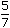# Aptitude - Time and Distance

Exercise : Time and Distance - General Questions
6.
In a flight of 600 km, an aircraft was slowed down due to bad weather. Its average speed for the trip was reduced by 200 km/hr and the time of flight increased by 30 minutes. The duration of the flight is:
1 hour
2 hours
3 hours
4 hours
Explanation:

Let the duration of the flight be x hours.

 Then, 600 - 600 = 200 x x + (1/2)600 - 1200 = 200 x 2x + 1x(2x + 1) = 32x2 + x - 3 = 0(2x + 3)(x - 1) = 0x = 1 hr.      [neglecting the -ve value of x]

7.
A man complete a journey in 10 hours. He travels first half of the journey at the rate of 21 km/hr and second half at the rate of 24 km/hr. Find the total journey in km.
220 km
224 km
230 km
234 km
Explanation:

 (1/2)x + (1/2)x = 10 21 24x + x = 20 21 2415x = 168 x 20x =168 x 20= 224 km. 15

8.
The ratio between the speeds of two trains is 7 : 8. If the second train runs 400 km in 4 hours, then the speed of the first train is:
70 km/hr
75 km/hr
84 km/hr
87.5 km/hr
Explanation:

Let the speed of two trains be 7x and 8x km/hr.

 Then, 8x =400= 100 4x =100= 12.5 8Speed of first train = (7 x 12.5) km/hr = 87.5 km/hr.

9.
A man on tour travels first 160 km at 64 km/hr and the next 160 km at 80 km/hr. The average speed for the first 320 km of the tour is:
35.55 km/hr
36 km/hr
71.11 km/hr
71 km/hr
Explanation:

 Total time taken =160 + 160hrs. = 9 hrs. 64 80 2Average speed =320 x 2km/hr = 71.11 km/hr. 9

10.
A car travelling withof its actual speed covers 42 km in 1 hr 40 min 48 sec. Find the actual speed of the car.
 17 6 km/hr 7
25 km/hr
30 km/hr
35 km/hrx =42 x 7 x 75= 35 km/hr. 5 x 126